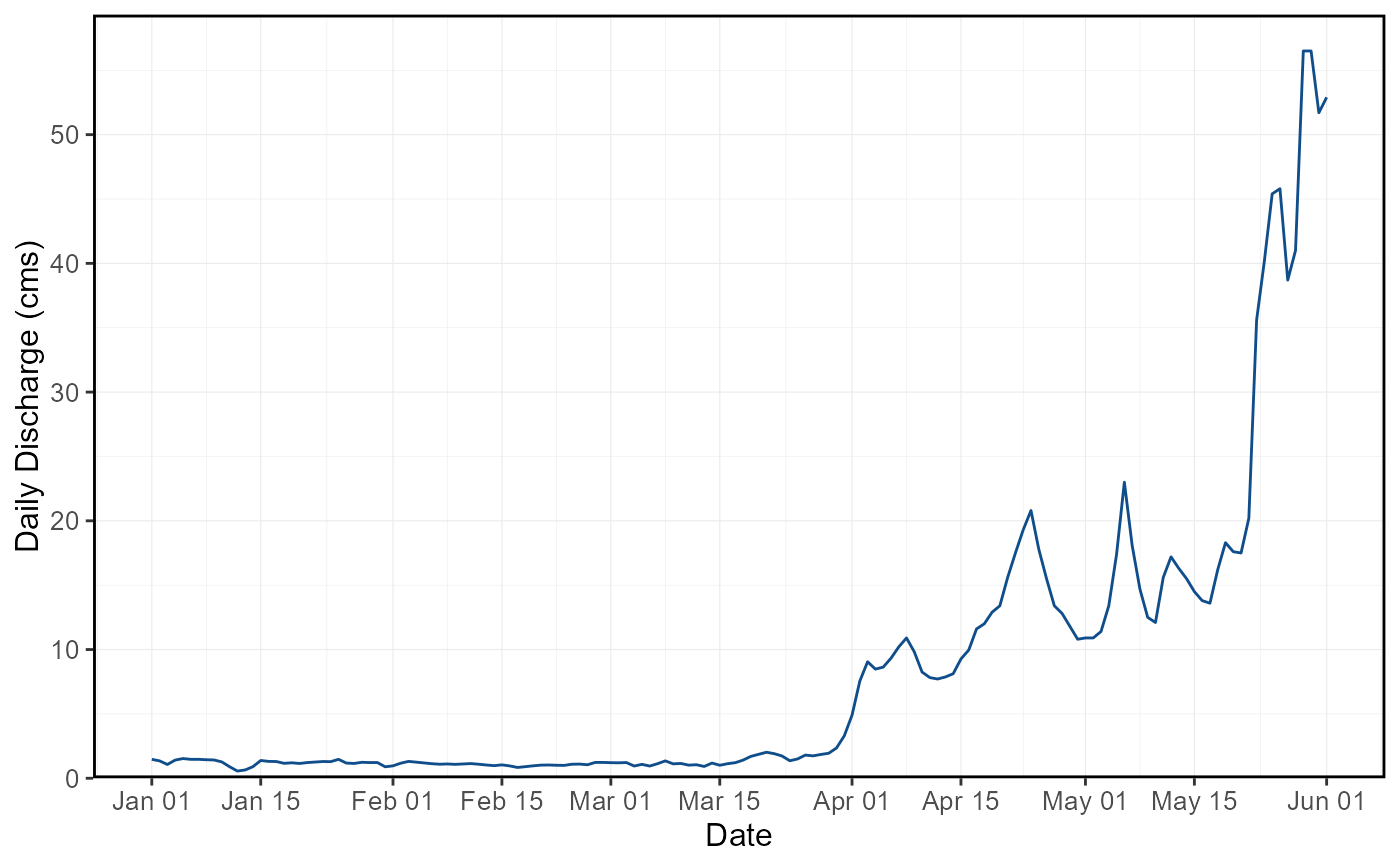Plot the daily mean flow values from a streamflow data set. Plots daily discharge values from all years, unless specified. Can choose specific dates to start and end plotting. Can choose to plot out each year separately. Multiple groups/stations can be plotted if provided with the groups argument. Returns a list of plots.

plot_flow_data(
data,
dates = Date,
values = Value,
groups = STATION_NUMBER,
station_number,
roll_days = 1,
roll_align = "right",
water_year_start = 1,
start_year,
end_year,
exclude_years,
start_date,
end_date,
log_discharge = FALSE,
plot_by_year = FALSE,
one_plot = FALSE,
include_title = FALSE
)

## Arguments

data Data frame of daily data that contains columns of dates, flow values, and (optional) groups (e.g. station numbers). Leave blank if using station_number argument. Name of column in data that contains dates formatted YYYY-MM-DD. Only required if dates column name is not 'Date' (default). Leave blank if using station_number argument. Name of column in data that contains numeric flow values, in units of cubic metres per second. Only required if values column name is not 'Value' (default). Leave blank if using station_number argument. Name of column in data that contains unique identifiers for different data sets, if applicable. Only required if groups column name is not 'STATION_NUMBER'. Function will automatically group by a column named 'STATION_NUMBER' if present. Remove the 'STATION_NUMBER' column beforehand to remove this grouping. Leave blank if using station_number argument. Character string vector of seven digit Water Survey of Canada station numbers (e.g. "08NM116") of which to extract daily streamflow data from a HYDAT database. Requires tidyhydat package and a HYDAT database. Leave blank if using data argument. Numeric value of the number of days to apply a rolling mean. Default 1. Character string identifying the direction of the rolling mean from the specified date, either by the first ('left'), last ('right'), or middle ('center') day of the rolling n-day group of observations. Default 'right'. Numeric value indicating the month (1 through 12) of the start of water year for analysis. Default 1. Numeric value of the first year to consider for analysis. Leave blank to use the first year of the source data. Numeric value of the last year to consider for analysis. Leave blank to use the last year of the source data. Numeric vector of years to exclude from analysis. Leave blank to include all years. Date (YYYY-MM-DD) of first date to consider for plotting. Leave blank if all years are required. Date (YYYY-MM-DD) of last date to consider for plotting. Leave blank if all years are required. Logical value to indicate plotting the discharge axis (Y-axis) on a logarithmic scale. Default TRUE. Logical value to indicate whether to plot each year of data individually. Default FALSE. Logical value to indicate whether to plot all groups/stations on one plot. Default FALSE. Logical value to indicate adding the group/station number to the plot, if provided. Default FALSE.

## Value

A ggplot2 object of daily flows from flow_data or HYDAT flow data provided

## Examples

# Run if HYDAT database has been downloaded (using tidyhydat::download_hydat())
if (file.exists(tidyhydat::hy_downloaded_db())) {

# Plot data from a data frame and data argument
flow_data <- tidyhydat::hy_daily_flows(station_number = "08NM116")
plot_flow_data(data = flow_data)

# Plot data directly from HYDAT
plot_flow_data(station_number = "08NM116")

# Plot statistics with custom years
plot_flow_data(station_number = "08NM116",
start_year = 1981,
end_year = 2010,
exclude_years = c(1991,1993:1995))

# Plot data between specific dates
plot_flow_data(station_number = "08NM116",
start_date = "1990-01-01",
end_date = "1990-06-01")

# Plot data multiple groups on one plot
plot_flow_data(station_number = c("08NM241", "08NM242"),
one_plot = TRUE)

}
#> Warning: Did not plot 3394 missing or excluded values between 1949-01-01 and 2017-12-31.#> Warning: Did not plot 3394 missing or excluded values between 1949-01-01 and 2017-12-31.#> Warning: Did not plot 1460 missing or excluded values between 1981-01-01 and 2010-12-31.#> Warning: Did not plot 328 missing or excluded values between 1983-01-01 and 2016-12-31.#> \$Daily_Flows#>